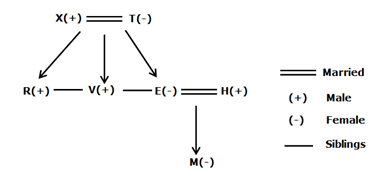# SBI Clerk Prelims Reasoning Ability Questions 2021 (Day-42)

Dear Aspirants, Our IBPS Guide team is providing new series of Reasoning Questions for SBI Clerk Prelims 2021 so the aspirants can practice it on a daily basis. These questions are framed by our skilled experts after understanding your needs thoroughly. Aspirants can practice these new series questions daily to familiarize with the exact exam pattern and make your preparation effective.

Start Quiz

Order and ranking

Direction (1-3): Study the following information carefully and answer the below questions.

There are five persons- H, M, W, Q and V with different weights. H is heavier thanonly one person. Only one person is weighted in between Q and W. W is lighter than V. M is neither theheaviest person nor the second heaviest person.

1) How many persons are heavier than V?

A.2

B.1

C.3

D.4

E.None

2) If the weight of H is 50kg, then what is the weight of M?

A.60 kg

B.65 kg

C.45 kg

D.55 kg

E.None of these

3) As many persons are lighter than Q is the same as heavier than ___?

A.M

B.H

C.W

D.V

E.None of these

Inequality

Direction (4-8): In each of the following questions, the relationship between different elements is shown in the statements followed by two conclusions. Find the conclusion which logically follows.

4) Statements:

M < F < H = O ;   L < K = T ≥ H; O > V = P

Conclusions:

I). L < P

II). F > T

A.If only conclusion I follows.

B.If only conclusion II follows.

C.If either conclusion I or II follows.

D.If neither conclusion I nor II follows.

E.If both conclusions I and II follow.

5) Statements:

M < A ≤ Q > Z > P; Y < T = F ≤ M

Conclusions:

I). Q > T

II). F < P

A.If only conclusion I follows.

B.If only conclusion II follows.

C.If either conclusion I or II follows.

D.If neither conclusion I nor II follows.

E.If both conclusions I and II follow.

6) Statements:

C < R < X = J < Z; Q > K > Z; E ≤ M > P < Q

Conclusions:

I). M ≤ Z

II). C < Q

A.If only conclusion I follows.

B.If only conclusion II follows.

C.If either conclusion I or II follows.

D.If neither conclusion I nor II follows.

E.If both conclusions I and II follow.

7) Statements:

B < C < T ≤ R = Q ;  F > P > G  = I > Q

Conclusions:

I). R < G

II). T < P

A.If only conclusion I follows.

B.If only conclusion II follows.

C.If either conclusion I or II follows.

D.If neither conclusion I nor II follows.

E.If both conclusions I and II follow.

8) Statements:

Q < X ≤ H ≤ K ≤ M; W ≥ L = E ≥ M

Conclusions:

I).  X < L

II). X = L

A.If only conclusion I follows.

B.If only conclusion II follows.

C.If either conclusion I or II follows.

D.If neither conclusion I nor II follows.

E.If both conclusions I and II follow.

Blood relation

Direction (9-10): Study the following information carefully and answer the below questions.

A family consists of seven members and two married couples. E is the only daughter of T who is the mother of V. H is the brother-in-law of R. M is the niece of R. X and T are a married couple. H and R are the same genders. H is the son-in-law of X.

9) How V is related to M?

A.Uncle

B.Aunty

C.Brother-in-law

D.Sister-in-law

E.Can’t be determined

10) Who among the following is the brother of E?

A.V

B.R

C.H

D.Both a and b

E.Both b and c

Directions (1-3) :

Q > V > W > H > M

Directions (9-10):